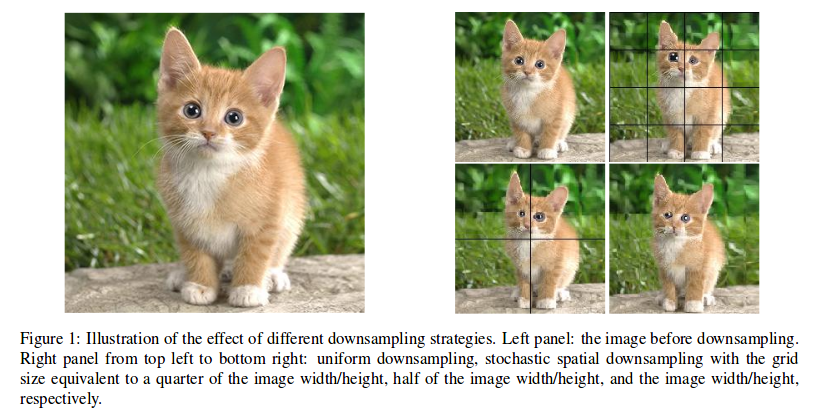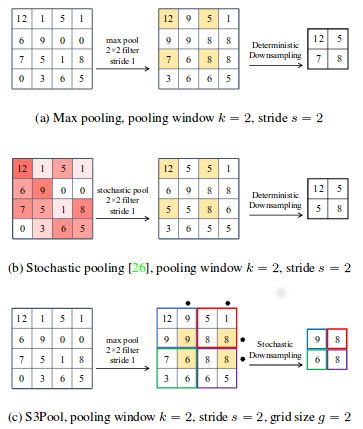# Idea

New kind of pooling aiming to learn from the information that is discarded by classical pooling. The image is spatially partioned and the rows and columns of a pooling grid are non-uniformly and stochastically chosen without replacement. It acts as a strong regularizer equivalent to implicit data augmentation because the network will see a different distorsion of the feature maps at each pass.

# Method

1. Partition the image $$(h,w)$$ into strips of size $$g$$ (both vertically and horizontally)
2. Randomly select $$\frac{g}{s}$$ rows and columns from each strip to obtain the downsampling grid -> The resulting feature map is of size $$\frac{h}{s}$$, $$\frac{w}{s}$$ (like a pooling with a window of size $$s$$) -> The pooling is likely to be non-uniform (different at each pass for the same image)
3. At test time, it is replaced either by standard max pooling or average pooling

# Application

Tested on CIFAR10-100 and STL-10 with ResNet

This method performs better than dropout and classical stochastic pooling both with and without data augmentation.

The training time goes up by about 10-15%

Downsampling : regular and stochastic spatial with different grid sizesPooling strategies :